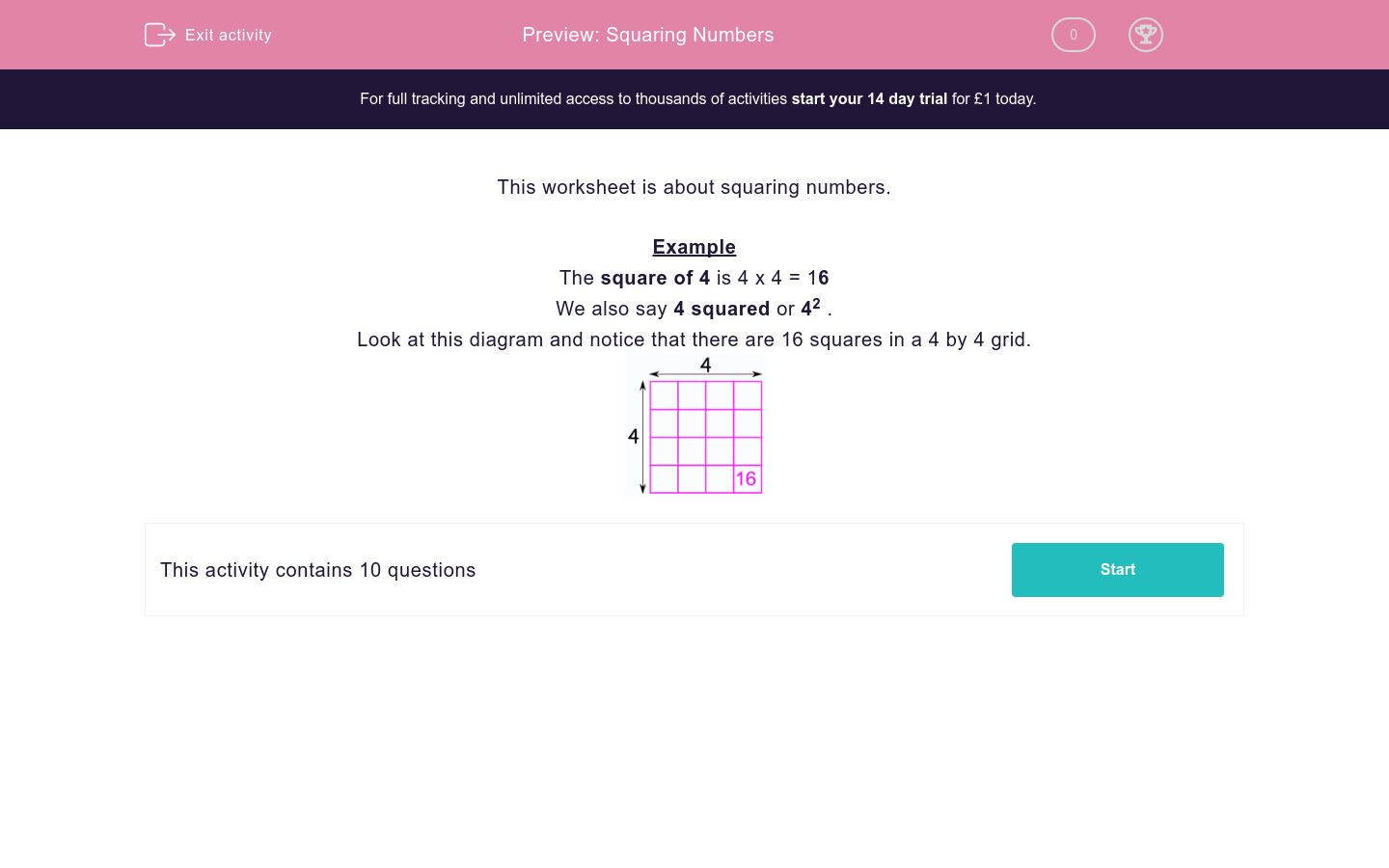# Squaring Numbers

In this worksheet, students practise squaring simple numbers and become familiar with the terminology of squaring numbers.Key stage:  KS 2

Curriculum topic:   Maths and Numerical Reasoning

Curriculum subtopic:   Square Numbers

Difficulty level:#### Worksheet Overview

This worksheet is about squaring numbers.

Example

The square of 4 is 4 x 4 = 16

We also say 4 squared or 42 .

Look at this diagram and notice that there are 16 squares in a 4 by 4 grid.### What is EdPlace?

We're your National Curriculum aligned online education content provider helping each child succeed in English, maths and science from year 1 to GCSE. With an EdPlace account you’ll be able to track and measure progress, helping each child achieve their best. We build confidence and attainment by personalising each child’s learning at a level that suits them.

Get started